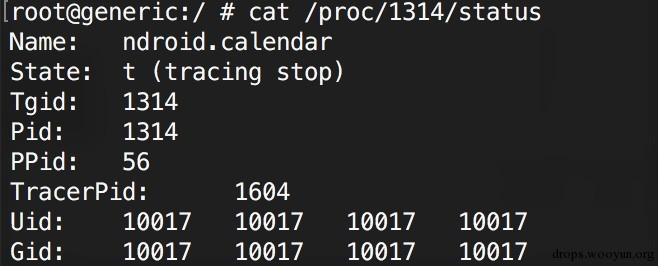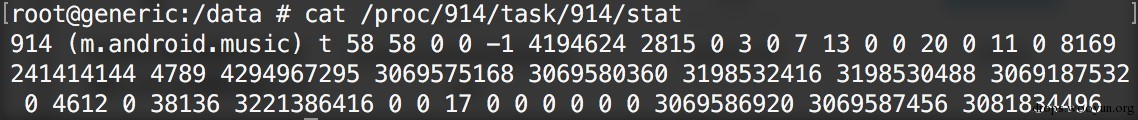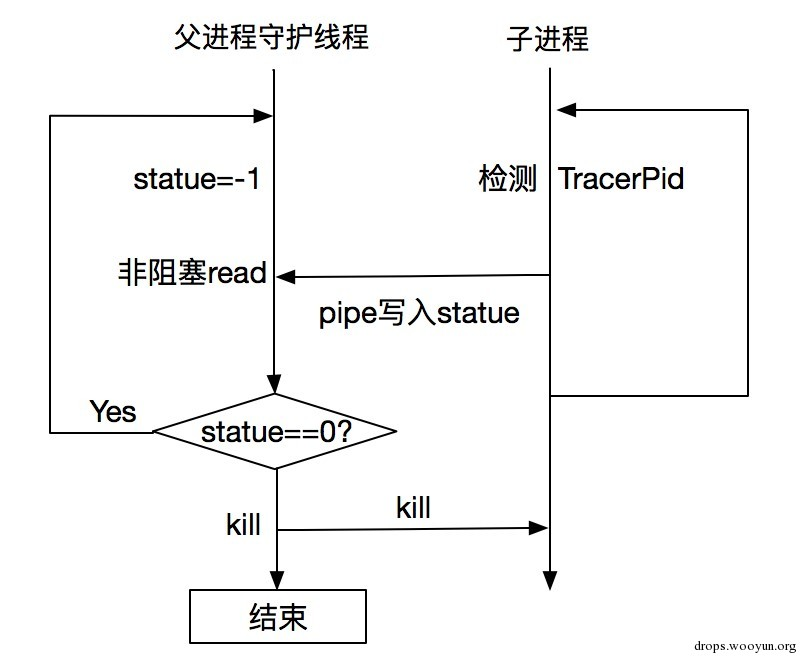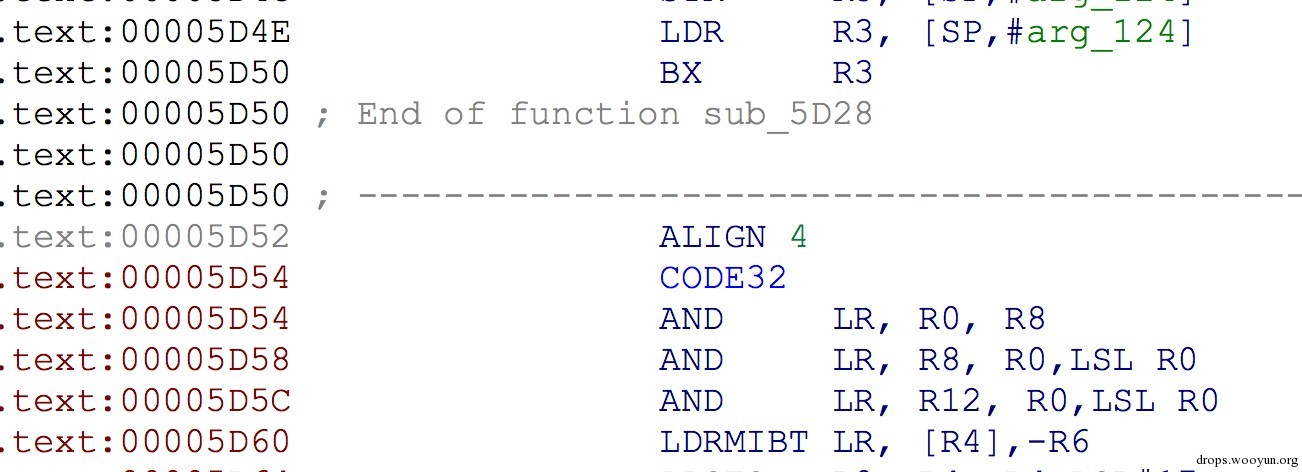Author：超六、曲和

# 0x01 检测关键文件

（1）/proc/pid/status、/proc/pid/task/pid/status（2）/proc/pid/stat、/proc/pid/task/pid/stat（3）/proc/pid/wchan、/proc/pid/task/pid/wchan

# 0x04 signal

1. 保存目标地址上的数据
2. 将目标地址上头几个字节替换成arm/thumb下的breakpoint指令

Arm架构下各类指令集breakpoint机器码如下：

Arm 0x01, 0x00, 0x9f, 0xef
Thumb 0x01, 0xde
Thumb2 0xf0, 0xf7, 0x00, 0xa0

1. 在目标地址上用原来的指令替换之前的breakpoint指令
2. 回退被跟踪进程的当前pc值

``````#!cpp
char dynamic_ccode[] = {0x1f,0xb4, //push {r0-r4}
0x01,0xde, //breakpoint
0x1f,0xbc, //pop {r0-r4}
0xf7,0x46};//mov pc,lr

char *g_addr = 0;

void my_sigtrap(int sig){

char change_bkp[] = {0x00,0x46}; //mov r0,r0
memcpy(g_addr+2,change_bkp,2);
__clear_cache((void*)g_addr,(void*)(g_addr+8)); // need to clear cache
LOGI("chang bpk to nop\n");

}

void anti4(){//SIGTRAP

int ret,size;
char *addr,*tmpaddr;

signal(SIGTRAP,my_sigtrap);

addr = (char*)malloc(PAGESIZE*2);

memset(addr,0,PAGESIZE*2);
g_addr = (char *)(((int) addr + PAGESIZE-1) & ~(PAGESIZE-1));

LOGI("addr: %p ,g_addr : %p\n",addr,g_addr);

ret = mprotect(g_addr,PAGESIZE,PROT_READ|PROT_WRITE|PROT_EXEC);
if(ret!=0)
{
LOGI("mprotect error\n");
return ;
}

size = 8;
memcpy(g_addr,dynamic_ccode,size);

__clear_cache((void*)g_addr,(void*)(g_addr+size)); // need to clear cache

__asm__("push {r0-r4,lr}\n\t"
"mov r0,pc\n\t"  //此时pc指向后两条指令
"add r0,r0,#4\n\t"//+4 是的lr 地址为 pop{r0-r5}
"mov lr,r0\n\t"
"mov pc,%0\n\t"
"pop {r0-r5}\n\t"
"mov lr,r5\n\t" //恢复lr
:
:"r"(g_addr)
:);

LOGI("hi, i'm here\n");
free(addr);

}
``````

``````#!cpp
struct r_debug {
int32_t r_version;
link_map_t* r_map;
void (*r_brk)(void);
int32_t r_state;
uintptr_t r_ldbase;
};
``````

r_debug是以静态变量的形式存在于linker中，其初始化代码如下：

``````#!cpp
static r_debug _r_debug = {1, NULL, &rtld_db_dlactivity, RT_CONSISTENT, 0};
``````

``````#!cpp
/*
* This function is an empty stub where GDB locates a breakpoint to get notified
* about linker activity.  It canʼt be inlined away, can't be hidden.
*/
extern "C" void __attribute__((noinline)) __attribute__((visibility("default"))) rtld_db_dlactivity() {
}
``````

# 0x05 检测软件断点

``````#!cpp
unsigned long GetLibAddr() {
unsigned long ret = 0;
char name[] = "libanti_debug.so";
char buf, *temp;
int pid;
FILE *fp;
pid = getpid();
sprintf(buf, "/proc/%d/maps", pid);
fp = fopen(buf, "r");
if (fp == NULL) {
puts("open failed");
goto _error;
}
while (fgets(buf, sizeof(buf), fp)) {
if (strstr(buf, name)) {
temp = strtok(buf, "-");
ret = strtoul(temp, NULL, 16);
break;
}
}
_error: fclose(fp);
return ret;
}

void anti5(){

Elf32_Ehdr *elfhdr;
Elf32_Phdr *pht;
unsigned int size, base, offset,phtable;
int n, i,j;
char *p;

//从maps中读取elf文件在内存中的起始地址
base = GetLibAddr();
if(base == 0){
LOGI("find base error\n");
return;
}

elfhdr = (Elf32_Ehdr *) base;

phtable = elfhdr->e_phoff + base;

for(i=0;i<elfhdr->e_phnum;i++){

pht = (Elf32_Phdr*)(phtable+i*sizeof(Elf32_Phdr));

if(pht->p_flags&1){
offset = pht->p_vaddr + base + sizeof(Elf32_Ehdr) + sizeof(Elf32_Phdr)*elfhdr->e_phnum;
LOGI("offset:%X ,len:%X",offset,pht->p_memsz);

p = (char*)offset;
size = pht->p_memsz;

for(j=0,n=0;j<size;++j,++p){

if(*p == 0x10 && *(p+1) == 0xde){
n++;
LOGI("### find thumb bpt %X \n",p);
}else if(*p == 0xf0 && *(p+1) == 0xf7 && *(p+2) == 0x00 && *(p+3) == 0xa0){
n++;
LOGI("### find thumb2 bpt %X \n",p);
}else if(*p == 0x01 && *(p+1) == 0x00 && *(p+2) == 0x9f && *(p+3) == 0xef){
n++;
LOGI("### find arm bpt %X \n",p);
}

}
LOGI("### find breakpoint num: %d\n",n);

}
}

}
``````

# 0x06 进程间通信

``````#!cpp
int pipefd;
int childpid;

void *anti3_thread(void *){

int statue=-1,alive=1,count=0;

close(pipefd);

while(read(pipefd,&statue,4)>0)
break;
sleep(1);

//这里改为非阻塞
fcntl(pipefd, F_SETFL, O_NONBLOCK); //enable fd的O_NONBLOCK

LOGI("pip-->read = %d", statue);

while(true) {

LOGI("pip--> statue = %d", statue);
read(pipefd, &statue, 4);
sleep(1);

LOGI("pip--> statue2 = %d", statue);
if (statue != 0) {
kill(childpid,SIGKILL);
kill(getpid(), SIGKILL);
return NULL;
}
statue = -1;
}
}

void anti3(){
int pid,p;
FILE *fd;
char filename[MAX];
char line[MAX];

pid = getpid();
sprintf(filename,"/proc/%d/status",pid);// 读取proc/pid/status中的TracerPid
p = fork();
if(p==0) //child
{
close(pipefd); //关闭子进程的读管道
int pt,alive=0;
pt = ptrace(PTRACE_TRACEME, 0, 0, 0); //子进程反调试
while(true)
{
fd = fopen(filename,"r");
while(fgets(line,MAX,fd))
{
if(strstr(line,"TracerPid") != NULL)
{
int statue = atoi(&line);
LOGI("########## tracer pid:%d", statue);
write(pipefd,&statue,4);//子进程向父进程写 statue值

fclose(fd);

if(statue != 0)
{
return ;
}

break;
}
}
sleep(1);

}
}else{
childpid = p;
}
}
pipe(pipefd);
pthread_create(&id_0,NULL,anti3_thread,(void*)NULL);
anti3();
``````# 0x07 dalvik 虚拟机内部相关字段

``````#!cpp
struct DvmGlobals {
…
bool   debuggerConnected;    /* debugger or DDMS is connected */
bool   debuggerActive;        /* debugger is making requests */
…
}
``````

``````#!cpp
/*
* static boolean isDebuggerConnected()
*
* Returns "true" if a debugger is attached.
*/
static void Dalvik_dalvik_system_VMDebug_isDebuggerConnected(const u4* args, JValue* pResult)
{
UNUSED_PARAMETER(args);
RETURN_BOOLEAN(dvmDbgIsDebuggerConnected());
}
``````

``````#!cpp
bool dvmDbgIsDebuggerConnected()
{
return gDvm.debuggerActive;
}
``````

# 0x08 IDA arm、thumb指令识别缺陷bx r3指令会切换指令集，而参数r3是动态计算出来的，IDA无法失败r3的值，而默认将bx r3后面的指令当成跳转地址，将后面地址的指令识别成了arm指令，而实际上其仍为thumb指令。

# 0x09 Ptrace

Ptrace是gdb等调试器实现的核心，通过ptrace可以监控、控制被调试进程的状态、信号、执行等。而每个进程在同一时刻最多只能被一个调试进程ptrace，根据这个原理，可以主动ptrace自己的关键子进程，这样可以在一定程度上防止子进程被调试。

# 0x0C Reference[微信] 扫描二维码打赏[支付宝] 扫描二维码打赏